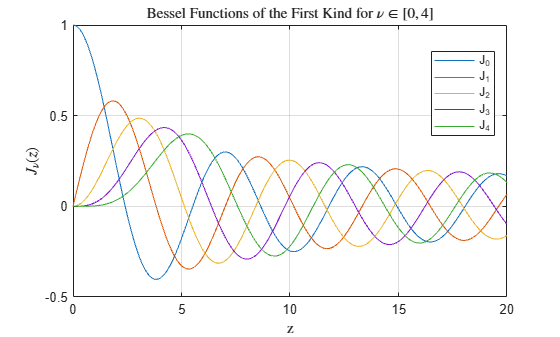# besselj

Bessel function of first kind

## Syntax

J = besselj(nu,Z)
J = besselj(nu,Z,scale)

## Description

example

J = besselj(nu,Z) computes the Bessel function of the first kind Jν(z) for each element in array Z.

example

J = besselj(nu,Z,scale) specifies whether to exponentially scale the Bessel function of the first kind to avoid overflow or loss of accuracy. If scale is 1, then the output of besselj is scaled by the factor exp(-abs(imag(Z))).

## Examples

collapse all

Define the domain.

z = 0:0.1:20;

Calculate the first five Bessel functions of the first kind. Each row of J contains the values of one order of the function evaluated at the points in z.

J = zeros(5,201); for i = 0:4 J(i+1,:) = besselj(i,z); end

Plot all of the functions in the same figure.

plot(z,J) grid on legend('J_0','J_1','J_2','J_3','J_4','Location','Best') title('Bessel Functions of the First Kind for $\nu \in [0, 4]$','interpreter','latex') xlabel('z','interpreter','latex') ylabel('$J_\nu(z)$','interpreter','latex')Calculate the unscaled (J) and scaled (Js) Bessel function of the first kind ${\mathit{J}}_{2}\left(\mathit{z}\right)$ for complex values of $\mathit{z}$.

x = -10:0.3:10; y = x'; z = x + 1i*y; scale = 1; J = besselj(2,z); Js = besselj(2,z,scale);

Compare the plots of the imaginary part of the scaled and unscaled functions. For large values of abs(imag(z)), the unscaled function quickly overflows the limits of double precision and stops being computable. The scaled function removes this dominant exponential behavior from the calculation, and thus has a larger range of computability compared to the unscaled function.

surf(x,y,imag(J)) title('Bessel Function of the First Kind','interpreter','latex') xlabel('real(z)','interpreter','latex') ylabel('imag(z)','interpreter','latex')surf(x,y,imag(Js)) title('Scaled Bessel Function of the First Kind','interpreter','latex') xlabel('real(z)','interpreter','latex') ylabel('imag(z)','interpreter','latex')## Input Arguments

collapse all

Equation order, specified as a scalar, vector, matrix, or multidimensional array. nu is a real number that specifies the order of the Bessel function of the first kind. nu and Z must be the same size, or one of them can be scalar.

Example: besselj(3,0:5)

Data Types: single | double

Functional domain, specified as a scalar, vector, matrix, or multidimensional array. besselj is real-valued where Z is positive. nu and Z must be the same size, or one of them can be scalar.

Example: besselj(1,[1-1i 1+0i 1+1i])

Data Types: single | double
Complex Number Support: Yes

Toggle to scale function, specified as one of these values:

• 0 (default) — No scaling

• 1 — Scale the output of besselj by exp(-abs(imag(Z)))

On the complex plane, the magnitude of besselj grows rapidly as the value of abs(imag(Z)) increases, so exponentially scaling the output is useful for large values of abs(imag(Z)) where the results otherwise quickly lose accuracy or overflow the limits of double precision.

Example: besselj(3,0:5,1)

collapse all

### Bessel Functions

This differential equation, where ν is a real constant, is called Bessel's equation:

${z}^{2}\frac{{d}^{2}y}{d{z}^{2}}+z\frac{dy}{dz}+\left({z}^{2}-{\nu }^{2}\right)y=0.$

Its solutions are known as Bessel functions.

The Bessel functions of the first kind, denoted Jν(z) and Jν(z), form a fundamental set of solutions of Bessel's equation for noninteger ν. Jν(z) is defined by

${J}_{\nu }\left(z\right)={\left(\frac{z}{2}\right)}^{\nu }\sum _{\left(k=0\right)}^{\infty }\frac{{\left(\frac{-{z}^{2}}{4}\right)}^{k}}{k!\Gamma \left(\nu +k+1\right)}\text{\hspace{0.17em}}.$

The Bessel functions of the second kind, denoted Yν(z), form a second solution of Bessel's equation that is linearly independent of Jν(z). Yν(z) is defined by

${Y}_{\nu }\left(z\right)=\frac{{J}_{\nu }\left(z\right)\mathrm{cos}\left(\nu \pi \right)-{J}_{-\nu }\left(z\right)}{\mathrm{sin}\left(\nu \pi \right)}\text{\hspace{0.17em}}.$

You can calculate Bessel functions of the second kind using bessely.

## Tips

The Bessel functions are related to the Hankel functions, also called Bessel functions of the third kind:

$\begin{array}{l}{H}_{\nu }^{\left(1\right)}\left(z\right)={J}_{\nu }\left(z\right)+i\text{\hspace{0.17em}}{Y}_{\nu }\left(z\right)\\ {H}_{\nu }^{\left(2\right)}\left(z\right)={J}_{\nu }\left(z\right)-i\text{\hspace{0.17em}}{Y}_{\nu }\left(z\right).\end{array}$

${H}_{\nu }^{\left(K\right)}\left(z\right)$ is besselh, Jν(z) is besselj, and Yν(z) is bessely. The Hankel functions also form a fundamental set of solutions to Bessel's equation (see besselh).